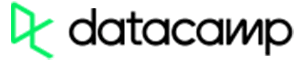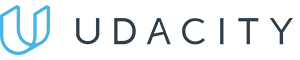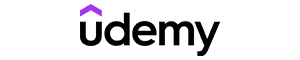Code has been added to clipboard!

# Importing and Using the Python Time Module

Published Feb 12, 2020
Updated Feb 12, 2020

TL;DR – The Python time module lets you represent time in objects, numbers, and strings, as well as perform other useful tasks.

## Preparing to work with Python time

To be able to work with time in Python, you need to first import the module:

``import time``

Python `time` module allows you to work with all time-related functions. Most of them simply call the platform C library functions that have the same name. However, there might be small differences between different platforms. The `time` module is available in all versions of Python.

## Understanding time in Python: the epoch

The most basic function in the Python `time` module is `time()`:

Example
``````seconds = time.time()
print("It's", seconds, "seconds since the epoch.")``````

It returns a floating point value that represents the number of seconds that have passed since the epoch. The epoch is a a platform-dependent point where the time starts.

If you're unsure what is the epoch on the system you're using, use the `gmtime()` function. It takes one argument (a number of seconds) and converts the time to a struct. If you define the number as zero, it will simply display the beginning of the epoch:

Example
``print(time.gmtime(0))``Pros
• Easy to use with a learn-by-doing approach
• Offers quality content
• Gamified in-browser coding experience
• The price matches the quality
• Suitable for learners ranging from beginner to advanced
Main Features
• Free certificates of completion
• Focused on data science skills
• Flexible learning timetablePros
• Simplistic design (no unnecessary information)
• High-quality courses (even the free ones)
• Variety of features
Main Features
• Nanodegree programs
• Suitable for enterprises
• Paid Certificates of completionPros
• Easy to navigate
• No technical issues
• Seems to care about its users
Main Features
• Huge variety of courses
• 30-day refund policy
• Free certificates of completion

## Converting Python time

The `gmtime()` function returns the struct time in Coordinated Universal Time (UTC). If you need it in local time, use `localtime()`. To get an opposite result, use `mktime()`:

Example
``````t = time.localtime(seconds)
print("The struct_time is:", t)

sec = time.mktime(t)
print("The number of seconds is:", sec)``````

If you need the Python time represented in a string, use `asctime()` (if you have a struct time) or `cttime()` (if you have a floating point value). Both of these functions will return a Python timestamp:

Example
``````t = (2020, 1, 13, 13, 18, 9, 0, 3, 0)
date = time.asctime(t)

seconds = time.time()
date2 = time.ctime(seconds)``````

## Using the Python time.sleep() function

The `time` module also allows you to time the execution of your threads. To delay a certain process, use the Python `time.sleep()` function:

Example
``````print("Hello.")
time.sleep(3)
print("It's been three seconds since we said hello.")``````

You only need to define one argument, which is a floating-point number. It represents the number of seconds to delay the action for.

Using the Python `time.sleep()` function with a while loop, you can also create a basic digital clock:

Example
``````while True:
localtime = time.localtime()
result = time.strftime("%I:%M:%S", localtime)
print(result)
time.sleep(1)``````

## Python time: useful tips

• There are two more Python modules that help you deal with time and date: `calendar` handles the calendar-related functions, and `datetime` lets you manipulate Python timestamps. You can also extend `datetime` withe the `dateutil` package from PyPI.
• If you're working with multithreaded programs, remember that the Python `time.sleep()` function only delays a single thread and not the whole process.

Days
Hours
Minutes
Seconds